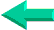# VBA Lesson 21: Functions in VBA for Excel

There are three topics in this lesson:
- using Excel functions within macros,
- using VBA functions within macros,
- creating new Excel functions with VBA.

Excel Functions

Some of the functions that you find in Excel are available through macros in this form:
Range ("C1").Value= Application.WorksheetFunction.Sum(Range("A1:A32"))
this sentence sums the values of cell A1 to A32 and stores the total in cell C1.

VBA Functions

Here are two  VBA functions that you will use  within your  Excel macros:

LCase, UCase
The " If" statements are case sensitive. When you test a string of characters and you do not know if the user will enter upper case or lower case letters, use the LCase or UCase functions within your " If" statement so that however the user enters his answer the statement will work.

If LCase(Selection.Value)= "yes" then...
or
If UCase(Selection.Value)= "YES" then...

Go to the next lesson
Lesson
22 : External Data and SQL in VBA for ExcelBack home